# C.1. Sum Notation

Sums can be concisely written using the upper-case Greek letter sigma. For example,is read, "The sum, for i from 1 to 4, of 2i2." This is: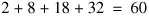Often the upper limit of the index (written above the sigma) is some other variable involved in the analysis, such as n. For example, in Section C.3, we will see a formula for the sum of the first n positive integers: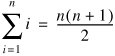In order to wrangle an expression into a form for which we have a formula, it is often useful to add or remove terms. For example:Substitution of variables is another useful technique. For example, to evaluate the sum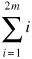we define n = 2m. Then: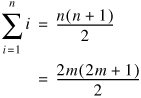Sum notation is sometimes used more casually. For example, to describe the total value of all of the cars on a sales lot, we might write: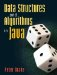Data Structures and Algorithms in Java
ISBN: 0131469142
EAN: 2147483647
Year: 2004
Pages: 216
Authors: Peter Drake

Similar book on Amazon

Flylib.com © 2008-2020.
If you may any questions please contact us: flylib@qtcs.net